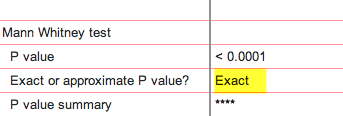KNOWLEDGEBASE - ARTICLE #1983

# Fixed bugs in the Mann-Whitney and Wilcoxon tests in Prism 6

## Mann-Whitney exact P values are a tiny bit wrong.

This bug occurs only for samples without ties, and when Prism computes an exact P value. This bug occurred in 6.00-6.05 and 6.0a-6.0f, and was fixed in 6.06 and 6.0g.The bug affects computation of the P value. The reported P value value was always smaller than it should be.

The bug appears about two-thirds of the time, but is tiny.  How tiny? For all P reported P values:

• <  0.0001: P value correct up to four digits in about ~44% cases
• < 0.001:   P value correct up to three digits n about ~60% cases
• < 0.01:     P value correct up to two digits in about ~90% cases

For p values less than 0.1 (when you most care), the average discrepancy between the reported and true p value is only ~0.0003, and the discrepancy  exceeds 0.001 only about 5% of the time.

If you reported that a difference was "statistically significant" (P < 0.05) there was a ~ 0.37% chance that the true P value is actually greater than 0.05 so your conclusion was wrong. This assumes that your data had no ties, and the P value was computed exactly, the conditions where the bug appears.  If your threshold was P<0.01, the error rate was about 0.1%.

For larger P values, the discrepancy is larger (but when P values are large, it is rare to care much about exactly what the value is).  The discrepancy was largest (but still small) when the two sample sizes were quite different from one another.

## Mann-Whitney test large P values

If there are no ties, and the correct one-tailed p value is 0.5, Prism incorrectly reports it as 1.0. If the correct two-tailed p value equals 1.0 it is correctly reported as ">0.9999" in the Mann-Whitney results, but incorrectly reported as 2.0 in a Monte Carlo analysis of the Mann Whitney test.  This was fixed in 6.02 and 6.0c.

The bug is in 6.00, 6.01, 6.0a and 6.0b, and fixed in 6.02 and 6.0c.

Also note that we have changed the algorithms used to cope with ties.

## Mann-Whitney test with large samples with ties.

When the total sample size exceeds 1290 and when at least two of those values are identical to each other (tied),  the results of the Mann-Whitney test from Prism 6.0a Mac was wrong. The correction for ties uses the expression N^3, where N is the total sample size.  When N>1290, that led to a math overflow error on the Mac. The Windows compiler automatically converts N from integer to real so there is no overflow error. Only in 6.0a. Fixed in 6.0b.

## Wilcoxon  matched pairs test in Prism 6 using Pratt's method

A new feature in Prism 6 is that we offer two choices for how to deal with the situation in the matched pairs test when both values are the same so the difference is zero. New to Prism 6 is resolving this method with the method of Pratt. Unfortunately, a bug gives wrong answers for this test. If you choose the conventional method used in prior Prism versions instead (eliminate those pairs or values, which Prism does automatically) then the results are fine. Fixed in 6.03 and 6.0d.

## Mann-Whitney test with a huge number of rows can cause Prism to crash

Mann-Whitney test of data sets with more than 30,000 rows and lots of tied values crashed all releases of Prism 6. Fixed in 7.00 and 7.0a.

Fixed bugs in these tests in Prism 3-5

## Explore the Knowledgebase

Analyze, graph and present your scientific work easily with GraphPad Prism. No coding required.